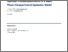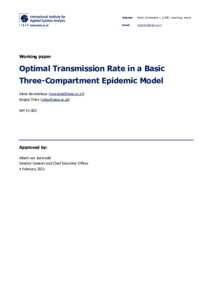# Optimal Transmission Rate in a Basic Three-Compartment Epidemic Model

& Orlov, S. (2021). Optimal Transmission Rate in a Basic Three-Compartment Epidemic Model. IIASA Working Paper. Laxenburg, Austria: WP-21-002Preview
Text
WP-21-002.pdf - Published Version

## Abstract

In this paper, we consider a communicable disease whose dynamics is described by a simple three-compartment epidemic model. The epidemic spread begins with a small number of infected persons brought into a totally susceptible population. We assume that the central planner can introduce policy measures to reduce the transmission rate and hence the basic reproduction number over some time interval in order to minimize the total number of infected individuals over the entire time horizon of the model. Our model is arguably the simplest possible model that can describe an epidemic spread and its control. This enables analytical treatment of the model and the derivation of the closed-form solution. We make the following conclusions. We observe that the total number of infected individuals depends only on the lowered basic reproduction number and the increment in the number of recovered individuals over the period of the policy measures’ application; it does not depend, for example, on the number of infected or recovered at the time of the policy inception. Since our model does not include policy costs, we obtain that in order to minimize the total number of infected individuals, the central planner should impose infinitely long policy measures. There is a set of optimal measures: Measures from this set are characterized by the tradeoff between the lowered basic reproduction number and the inception time of the measures. Since infinitely long application of measures is likely to be infeasible in the real world, we derive measures of finite duration which are near-to-optimal, that is measures which lead to the total number of infected individuals that is higher than the optimal number by a small positive ε. Our analysis of these near-to-optimal measures suggests that a robust way to ensure a reasonable duration of measures and at the same time to achieve that they indeed lead to an ε-optimal outcome is as follows. The central planner could choose the optimal policy inception time and the optimal lowered basic reproduction number, and then keep these measures for as long as it is feasible. The duration of this period will determine the number of infected individuals that will be achieved and the corresponding ε.

Item Type: Monograph (IIASA Working Paper) Advancing Systems Analysis (ASA)Advancing Systems Analysis (ASA) > Exploratory Modeling of Human-natural Systems (EM) Luke Kirwan 08 Feb 2021 08:15 27 Aug 2021 17:34 http://pure.iiasa.ac.at/17030View Item